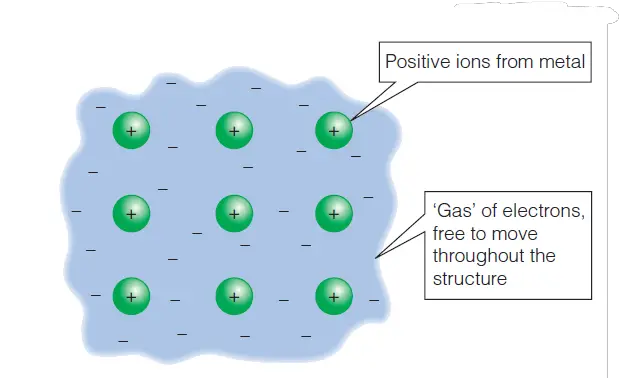# Resistance of metal increases with an increase in temperature – how?

When current flows through the material of a circuit, such as the metal of the connecting wires, the material of the circuit gets in the way of the flow of the charge. On a microscopic level, as the electrons flow through the metal they collide with the vibrating positive ion cores of the metal structure.

The collisions between the electrons and the positive ion cores transfer electrical energy from the electrons to the structure of the metal, causing the metal ion cores to vibrate more, thus heating up the wire.

The opposition of a component to the flow of electric current through it is called resistance, (symbol R, unit ohms, Ω). Now, let’s see how the Resistance of metal increases with an increase in temperature.

## Resistance of metal increases with an increase in temperature – how?figure 1: Resistance of metal increases with an increase in temperature – how? The structure of a metal explains what happens to the resistance of the metal when it is heated

The model of a metal shown above explains what happens to the resistance of the metal when it is heated. The vibrating, positively charged, ion cores in a metal conductor move around much more as the temperature increases, thus getting in the way of electron flow. This opposes the flow of the electrons moving through the structure. Thus, the resistance of a metal increases as the temperature increases.

Components with very high resistances let very little current through them and are considered to be electrical insulators. Some materials, at very low temperatures, have zero resistance. These materials are called superconductors.

Resistance of metal increases with an increase in temperature – how?
Scroll to top
error: physicsTeacher.in